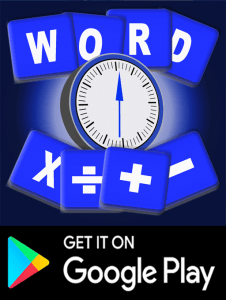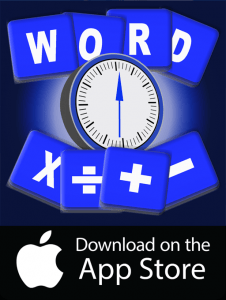# Mental Math Tips

Mental math tips – keep your brain tuned.

I watch the lovely Lily on SBS’s Letters and Numbers. She amazes and impresses with the seemingly effortless way she arrives at a correct answer to a calculation. It seems to take no time at all. SBS’s show is based on the United Kingdom’s Countdown. In both shows contestants compete to outdo an opponent in producing the longest word from a group of letters. Also they must arrive at a given number by using calculations with six numbers.

I can sometimes arrive at a correct answer. Using the six numbers and brackets and operators +, * and minus I eventually set there.but it takes me too long. Remember you need to use BODMAS to calculate in the correct order.  Click on link here to find out more about BODMAS. I need to be faster. How can I improve my mental maths skills?

## Strategies

### 1  Use a calculator less

It is possible to improve by using a few strategies. Many of us have become lazy by using calculators when we could quite easily work out a simple sum. By not testing ourselves and not using resources we used to use, we have lost some of the edge we once had.

Calculators are invaluable in some circumstances but we should not need them to calculate a 15% discount on an airfare for instance.The airfare from Cairns to Brisbane is \$360. We are told there is  a 15% discount for an early booking. It is easy to work out 10% would be \$36 and 5% would be \$18. Therefore the new fare would be \$360 – \$44 which equals \$316. It is often quicker to do the sum mentally but we have become lazy and untrusting of our own abilities.

Mental maths skills allow you to do an exact calculation in your head which saves time. Much more importantly they allow you to make an estimation. This gets you close enough to the correct answer in a multiple choice situation. It also protects you from making big mistakes with pen and paper such as having a decimal point in the wrong place.

Being adept at mental arithmetic is something you can attain. You just need to remember a few simple rules. Establishing good basic calculation skills makes all areas of mathematics easier and quicker to complete.

### 2  Add and Subtract From Left to Right

Remember how you were taught in school to add and subtract numbers from right to left (don’t forget to carry the one!)? That’s all fine and well when doing maths with pencil and paper. However when performing mental maths it’s better to do it moving from left to right. Switching the order so that you start with the largest values makes it a bit more intuitive. It is easier to figure out. So when adding 58 to 26, start with the first column and calculate 50+20=70, then 8+6=14, which added together is 84. Easy, peasy.

### 3 Mental math tips Make It Easy on Yourself

When confronted with a difficult calculation, try to find a way of simplifying the problem by temporarily shifting the values around. When calculating 593+680, for example, add 7 to 593 to get 600 (more manageable). Calculate 600+680, which is 1280, and then take away that additional 7 to get the correct answer, 1273.

You can do a similar thing with multiplication. For 89 x 6, calculate 90 x 6 instead, and then subtract that additional 6, so 540-6=534

### 4 Memorising multiplication tables helps improve mental math

This is an important aspect of mental maths, and it shouldn’t be discounted.

Spencer Greenberg, a mathematician and founder of ClearerThinking.org, says that by memorising these basic “building blocks” of maths, we can instantly get answers to simple problems that are embedded within more difficult ones. So if you’ve forgotten these tables, it would do you well to quickly brush up. While you’re at it, memorise your 1/n tables so you can quickly recall that 1/6 is 0.166, 1/3 is 0.333, and 3/4 is 0.75.

#### Mental math tips – Remember Cool Multiplication Tricks

To help you do simple multiplication, it’s important to remember some nifty tricks. One of the most obvious rules is that any number that’s multiplied by 10 just needs to have a zero placed at the end When multiplying by 5, your answer will always end in either a 0 or 5.

Also, when multiplying a number by 12, it’s always 10 times plus two times that number. For example, when calculating 12 x 4, do 4 x1 0=40, and 4 x 2=8, and then 40+8=48. One of my favourites is multiplying by 15: just multiply your number by 10, and then add half to the answer (e.g. 4 x 15 = 4 x 10=40, plus half that answer, 20, giving you 60).

There’s also a neat trick for multiplying by 16. First, multiply the number in question by 10, and then multiply half the number by 10. Then add those two results together with the number itself to get your final answer. So to calculate 16 x 24, first calculate 10 x 24 = 240, then figure out half of 24, which is 12, and multiply by 10, giving you 120. Simple maths finishes it up: 240 +120 + 24=384.

Similar tricks exist for other numbers, which you can read about here.

Practise not only up to your 12 times table but also 13, 15, 25, 125. You can practise these on our homework app.

### 5 Mental math tips Practise regularly

Give yourself a few calculations to do each day, starting easy and gradually getting harder.

When all’s said and done, if you really want to become better at maths, do more maths. Read, practice, become better. Because, according to research conducted by a Norway university, the only way to really become better at maths is to practise doing maths.

####